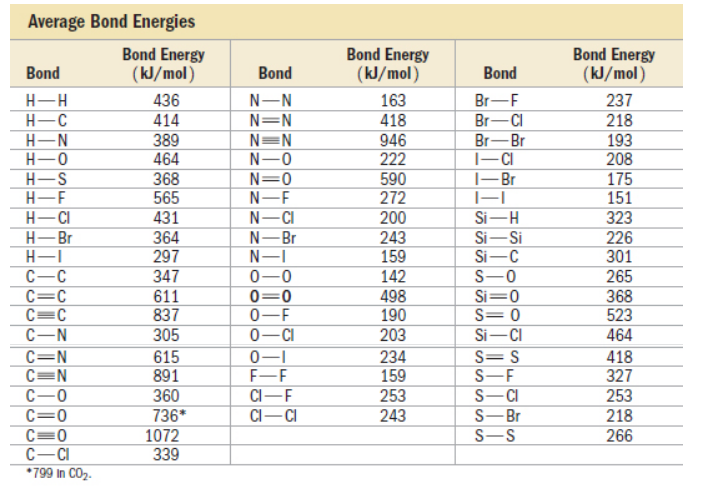Problem: Use bond energies to determine ΔH rxn for the reaction between ethanol and hydrogen chloride.CH3CH2OH(g) + HCl(g) → CH 3CH2Cl(g) + H2O(g)a. -12 kJb. 1549 kJc. 12 kJd. -1549 kJ

FREE Expert Solution
83% (426 ratings)
Problem Details

Use bond energies to determine ΔH rxn for the reaction between ethanol and hydrogen chloride.

CH3CH2OH(g) + HCl(g) → CH 3CH2Cl(g) + H2O(g)

a. -12 kJ

b. 1549 kJ

c. 12 kJ

d. -1549 kJWhat scientific concept do you need to know in order to solve this problem?

Our tutors have indicated that to solve this problem you will need to apply the Bond Energy concept. You can view video lessons to learn Bond Energy Or if you need more Bond Energy practice, you can also practice Bond Energy practice problems .

What is the difficulty of this problem?

Our tutors rated the difficulty of Use bond energies to determine ΔH rxn for the reaction betwe... as medium difficulty.

How long does this problem take to solve?

Our expert Chemistry tutor, Dasha took 8 minutes to solve this problem. You can follow their steps in the video explanation above.

What professor is this problem relevant for?

Based on our data, we think this problem is relevant for Professor Davis' class at UCF.